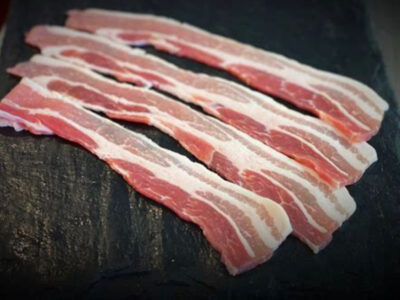Manuka Smoked Streaky Bacon Small Goods\$16 jQuery(function(\$){ var price = '16'; currency = '\$'; /* \$('[name=quantity]').change(function(){ if (!(this.value < 1)) { var product_total = parseFloat(price * this.value); \$(this).closest('.woocom-list-content').find('.product_total_price .price .woocommerce-Price-amount').html(currency + product_total.toFixed(2)); } }); jQuery( '.variations_form' ).each( function() { jQuery(this).on( 'found_variation', function( event, variation ) { var price = variation.display_price; var quantity = \$(this).find('[name=quantity]').val(); if (!(quantity < 1)) { console.log(price); var product_total = parseFloat(price * quantity); \$(this).closest('.woocom-list-content').find('.product_total_price .price .woocommerce-Price-amount').html(currency + product_total.toFixed(2)); } }); });*/ }); Free range streaky bacon, smoked over a natural Manuka woodfire. An essential for every Sunday morning cooked breakfast. 500g Manuka Smoked Streaky Bacon quantity ADD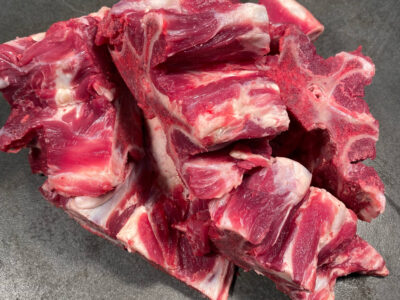Meaty Bones Pet Food\$9.00 jQuery(function(\$){ var price = '9.00'; currency = '\$'; /* \$('[name=quantity]').change(function(){ if (!(this.value < 1)) { var product_total = parseFloat(price * this.value); \$(this).closest('.woocom-list-content').find('.product_total_price .price .woocommerce-Price-amount').html(currency + product_total.toFixed(2)); } }); jQuery( '.variations_form' ).each( function() { jQuery(this).on( 'found_variation', function( event, variation ) { var price = variation.display_price; var quantity = \$(this).find('[name=quantity]').val(); if (!(quantity < 1)) { console.log(price); var product_total = parseFloat(price * quantity); \$(this).closest('.woocom-list-content').find('.product_total_price .price .woocommerce-Price-amount').html(currency + product_total.toFixed(2)); } }); });*/ }); Meaty Beef Spine Bone (Chuck Bone) Ideal for stocks & broths Meaty Bones quantity ADD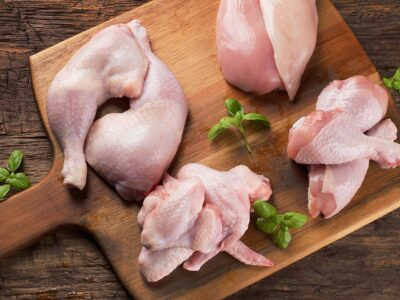Peri Peri Chicken Thighs Chicken\$12.5 jQuery(function(\$){ var price = '12.5'; currency = '\$'; /* \$('[name=quantity]').change(function(){ if (!(this.value < 1)) { var product_total = parseFloat(price * this.value); \$(this).closest('.woocom-list-content').find('.product_total_price .price .woocommerce-Price-amount').html(currency + product_total.toFixed(2)); } }); jQuery( '.variations_form' ).each( function() { jQuery(this).on( 'found_variation', function( event, variation ) { var price = variation.display_price; var quantity = \$(this).find('[name=quantity]').val(); if (!(quantity < 1)) { console.log(price); var product_total = parseFloat(price * quantity); \$(this).closest('.woocom-list-content').find('.product_total_price .price .woocommerce-Price-amount').html(currency + product_total.toFixed(2)); } }); });*/ }); Juicy boneless skinless chicken thighs marinated in a zesty hot Peri Peri sauce made in store 500g1 KG Peri Peri Chicken Thighs quantity ADD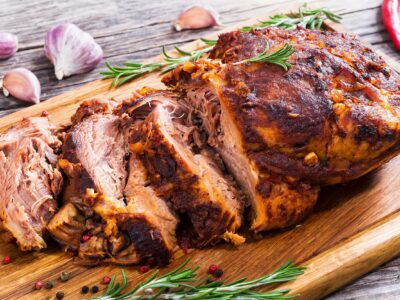Pork Loin Chops Pork\$11 jQuery(function(\$){ var price = '11'; currency = '\$'; /* \$('[name=quantity]').change(function(){ if (!(this.value < 1)) { var product_total = parseFloat(price * this.value); \$(this).closest('.woocom-list-content').find('.product_total_price .price .woocommerce-Price-amount').html(currency + product_total.toFixed(2)); } }); jQuery( '.variations_form' ).each( function() { jQuery(this).on( 'found_variation', function( event, variation ) { var price = variation.display_price; var quantity = \$(this).find('[name=quantity]').val(); if (!(quantity < 1)) { console.log(price); var product_total = parseFloat(price * quantity); \$(this).closest('.woocom-list-content').find('.product_total_price .price .woocommerce-Price-amount').html(currency + product_total.toFixed(2)); } }); });*/ }); 500g1 KG Pork Loin Chops quantity ADD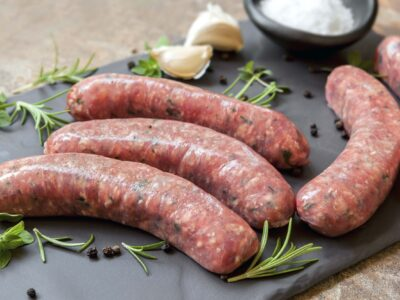Pork, Sweet Gala Apple & Sage Sausages Sausages\$13 jQuery(function(\$){ var price = '13'; currency = '\$'; /* \$('[name=quantity]').change(function(){ if (!(this.value < 1)) { var product_total = parseFloat(price * this.value); \$(this).closest('.woocom-list-content').find('.product_total_price .price .woocommerce-Price-amount').html(currency + product_total.toFixed(2)); } }); jQuery( '.variations_form' ).each( function() { jQuery(this).on( 'found_variation', function( event, variation ) { var price = variation.display_price; var quantity = \$(this).find('[name=quantity]').val(); if (!(quantity < 1)) { console.log(price); var product_total = parseFloat(price * quantity); \$(this).closest('.woocom-list-content').find('.product_total_price .price .woocommerce-Price-amount').html(currency + product_total.toFixed(2)); } }); });*/ }); New Zealands Gold Medal Gourmet Pork Sausage 2018/2019. Deliciously sweet and juicy sausage with a touch of sage. Medium grind, great flavour for the whole family. 6 Pack9 Pack12 Pack Pork, Sweet Gala Apple & Sage Sausages quantity ADDPure Beef Sausages Sausages\$11.5 jQuery(function(\$){ var price = '11.5'; currency = '\$'; /* \$('[name=quantity]').change(function(){ if (!(this.value < 1)) { var product_total = parseFloat(price * this.value); \$(this).closest('.woocom-list-content').find('.product_total_price .price .woocommerce-Price-amount').html(currency + product_total.toFixed(2)); } }); jQuery( '.variations_form' ).each( function() { jQuery(this).on( 'found_variation', function( event, variation ) { var price = variation.display_price; var quantity = \$(this).find('[name=quantity]').val(); if (!(quantity < 1)) { console.log(price); var product_total = parseFloat(price * quantity); \$(this).closest('.woocom-list-content').find('.product_total_price .price .woocommerce-Price-amount').html(currency + product_total.toFixed(2)); } }); });*/ }); A fine ground beef creating a beautiful bite on a classic Kiwi snag. 6 Pack9 Pack12 Pack Pure Beef Sausages quantity ADDPure Pork Sausages Sausages\$11.5 jQuery(function(\$){ var price = '11.5'; currency = '\$'; /* \$('[name=quantity]').change(function(){ if (!(this.value < 1)) { var product_total = parseFloat(price * this.value); \$(this).closest('.woocom-list-content').find('.product_total_price .price .woocommerce-Price-amount').html(currency + product_total.toFixed(2)); } }); jQuery( '.variations_form' ).each( function() { jQuery(this).on( 'found_variation', function( event, variation ) { var price = variation.display_price; var quantity = \$(this).find('[name=quantity]').val(); if (!(quantity < 1)) { console.log(price); var product_total = parseFloat(price * quantity); \$(this).closest('.woocom-list-content').find('.product_total_price .price .woocommerce-Price-amount').html(currency + product_total.toFixed(2)); } }); });*/ }); A fine ground pork creating a beautiful bite on a classic English banger. 6 Pack9 Pack12 Pack Pure Pork Sausages quantity ADD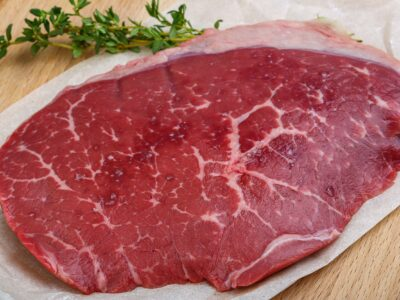Rolled Beef Beef\$20 jQuery(function(\$){ var price = '20'; currency = '\$'; /* \$('[name=quantity]').change(function(){ if (!(this.value < 1)) { var product_total = parseFloat(price * this.value); \$(this).closest('.woocom-list-content').find('.product_total_price .price .woocommerce-Price-amount').html(currency + product_total.toFixed(2)); } }); jQuery( '.variations_form' ).each( function() { jQuery(this).on( 'found_variation', function( event, variation ) { var price = variation.display_price; var quantity = \$(this).find('[name=quantity]').val(); if (!(quantity < 1)) { console.log(price); var product_total = parseFloat(price * quantity); \$(this).closest('.woocom-list-content').find('.product_total_price .price .woocommerce-Price-amount').html(currency + product_total.toFixed(2)); } }); });*/ }); Ideal for slow roasting and slow cooker 1 KG1.5 KG2 KG2.5 KG3 KG Rolled Beef quantity ADDRump Steak Beef\$13 jQuery(function(\$){ var price = '13'; currency = '\$'; /* \$('[name=quantity]').change(function(){ if (!(this.value < 1)) { var product_total = parseFloat(price * this.value); \$(this).closest('.woocom-list-content').find('.product_total_price .price .woocommerce-Price-amount').html(currency + product_total.toFixed(2)); } }); jQuery( '.variations_form' ).each( function() { jQuery(this).on( 'found_variation', function( event, variation ) { var price = variation.display_price; var quantity = \$(this).find('[name=quantity]').val(); if (!(quantity < 1)) { console.log(price); var product_total = parseFloat(price * quantity); \$(this).closest('.woocom-list-content').find('.product_total_price .price .woocommerce-Price-amount').html(currency + product_total.toFixed(2)); } }); });*/ }); Under rated frying steak, most flavoursome of all the tradition tender cuts. Speak to the team to discover their tips on getting a succulent juicy result 400g500g800g1 KG Rump Steak quantity ADD